# A perimeter

A perimeter of a parallelogram is 2.8 meters. The length of one of its sides is equal to one-seventh of the entire perimeter. Find lengths of the sides of the parallelogram.

a =  0.4 m
b =  1 m

### Step-by-step explanation: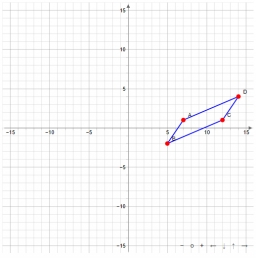Did you find an error or inaccuracy? Feel free to write us. Thank you!Tips to related online calculators
Need help to calculate sum, simplify or multiply fractions? Try our fraction calculator.
Check out our ratio calculator.

#### You need to know the following knowledge to solve this word math problem:

We encourage you to watch this tutorial video on this math problem:

## Related math problems and questions:

• ParallelogramThe parallelogram has sides lengths in the ratio 3: 4 and perimeter 2.8 meters. Determine the lengths of the sides.
• ParallelogramThe perimeter of the parallelogram is 417 cm. The length of one side is 1.7-times longer than the length of the shorter side. What is the length of sides of a parallelogram?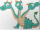Three sides of a quadrilateral are equal to the foreside which is 16 cm long. What is the length of the one with equal sides if the perimeter is 58 cm?
• The sidesThe sides of a rectangle are in a ratio of 2:3, and its perimeter is 1 1/4 inches. What are the lengths of its side? Draw it.
• Trapezoid RTThe plot has a shape of a rectangular trapezium ABCD, where ABIICD with a right angle at the vertex B. side AB has a length 36 m. The lengths of the sides AB and BC are in the ratio 12:7. Lengths of the sides AB and CD are a ratio 3:2. Calculate consumpti
• In aIn a triangle, the aspect ratio a: c is 3: 2, and a: b is 5: 4. The perimeter of the triangle is 74cm. Calculate the lengths of the individual sides.
• Similar trianglesThe triangles ABC and XYZ are similar. Find the missing lengths of the sides of the triangles. a) a = 5 cm b = 8 cm x = 7.5 cm z = 9 cm b) a = 9 cm c = 12 cm y = 10 cm z = 8 cm c) b = 4 cm c = 8 cm x = 4.5 cm z = 6 cm
• The parallelogram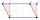The parallelogram has one side 2 dm long, which is one-sixth of its circumference. How many cm does the other side of the parallelogram measure?
• MidpointsTriangle ABC with sides a = 5 cm, b = 3 cm, c = 40mm has a midpoint of K, L, M. How many centimeters is long perimeter of parallelogram KBLM?
• Parallelogram perimeter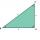The ABC triangle with sides a = 5cm, b = 3cm, c = 40mm has the center of the sides of the K,L,M. How many cm have the KBLM parallelogram perimeter?
• Ratio of sidesThe triangle has a circumference of 21 cm, and the length of its sides is in a ratio of 6: 5: 3. Find the length of the longest side of the triangle in cm.
• Find theFind the radius of the circular base of the vessel, whose perimeter is 2.51 m. Write the result to one decimal place in meters. Via the π sign - Ludolph's number
• OrchardRoute passes trapezoidal orchard perpendicular to the parallel sides. It is 80 cm wide. The lengths of the bases are in the ratio 5:3 and the length of the longer base to the length of the path is in the ratio 5:6. How many square meters occupies the rout
• ParallelogramCalculate the missing side of a parallelogram, if you know the perimeter o and one side: a) o = 7.2 cm; b = 1.8 cm b) o = 5.4 cm; a = 1.9 cm
• The gardenThe garden has the shape of a rectangular trapezium. The bases have lengths of 27 meters and 36 meters, the trapezoid's height is 12 meters. Calculate how much fence will cost this garden if one meter costs 1.5 €?
• Perimeter of a triangle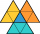If the perimeter of a triangle is 6 2/3 cm and the lengths of two of its sides are 2 1/2 cm and 3 1/3 cm, find the length if the third side
• Rectangular gardenThe sides of the rectangular garden are in ratio 1: 2. The diagonal has a length of 20 meters. Calculate the area and perimeter of the garden.